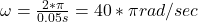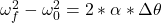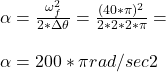Question

When it is at rotating at full speed, a disk drive in a certain old computer game system revolves once every 0.050 seconds. Starting from rest, it takes two revolutions for the disk to reach full speed. Assuming that the angular acceleration of the disk is constant, what is its angular acceleration while it is speeding up

1.Explanation:

• If the disk drive revolves once every 0.05 sec, this means that the angular velocity can be expressed as follows:• (just applying the definition of angular velocity)
• Now, it the disk started from rest, and took two revolutions to reach to full speed, assuming that the angular acceleration is constant, we can find the angular acceleration applying this kinematic equation:• where Δθ, is the angle rotated while it went from rest to full speed, i.e., 2 revolutions, or 2*π rads.
• Replacing by the value that we found for ωf (as ω₀ = 0), we  can solve for α :